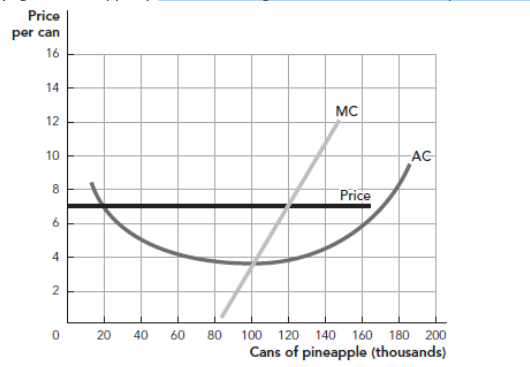# Priceper can1614MC1210ACPrice62206080 100 120 140 160 180 200Cans of pineapple (thousands)404t

Question
1 views

Refer to the figure. What is their total profit or loss?

–\$400,000

\$360,000

\$840,000

–\$200,000help_outlineImage TranscriptionclosePrice per can 16 14 MC 12 10 AC Price 6 2 20 60 80 100 120 140 160 180 200 Cans of pineapple (thousands) 40 4t fullscreen
check_circle

Step 1

The profit is the excess revenue made by the firm after deducting the total cost from the total revenue. Thus, it is the additional revenue made after covering the cost of production by a firm. The profit can be calculated by subtracting the total cost of production from the total revenue made by the firm.

Step 2

In this case, the price of the product is given to be \$7 per can and the quantity is determined at the point where the marginal cost curve intersects with the marginal revenue curve which is the horizontal straight price line and it is 120,000 cans. Thus, the total revenue of the firm can be calculated by multiplying the total quantity with the per unit price as follows:

Step 3

The average cost of producing a can is given to be approximately \$4 and thus, the total cost of producing the 12...

### Want to see the full answer?

See Solution

#### Want to see this answer and more?

Solutions are written by subject experts who are available 24/7. Questions are typically answered within 1 hour.*

See Solution
*Response times may vary by subject and question.
Tagged in